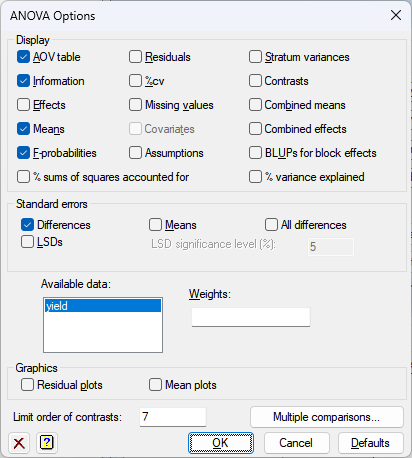1. Home
2. ANOVA Options

# ANOVA Options

Use this to select the output to be generated initially by ANOVA – the same information can also be printed after the analysis, using the Further Output dialog.## Display

Specifies which items of output are to be produced.

 AOV table Analysis of variance table Information Details of large residuals, non-orthogonality and any aliasing in the model Effects Tables of estimated treatment parameters Means Tables of means for treatment terms F-probabilities F-probabilities for the analysis of variance table % sums of squares accounted for This uses the A%VARIANCE procedure to calculate the percentage sums of squares accounted for by treatments in the strata Residuals Tables of estimated residuals %cv Coefficients of variation and standard errors of individual units Missing values Estimates of missing values Covariates Estimates of covariate regression coefficients Screening tests Screening tests for treatment terms (unbalanced treatment structure only) Assumptions Displays any warnings produced by homogeneity, normality and stability tests (not available for unbalanced treatment structure). See ACHECK procedure for details of the tests that are performed. Stratum variances Estimated variances of the units in each stratum Contrasts Estimated contrasts of treatment effects Combined means Means for treatment terms combining information from all the strata in which each term is estimated Combined effects Estimated effects of treatment terms combining information from all the strata in which each term is estimated BLUPs for block effects The best linear unbiased predictors (BLUPs) for block terms. These differ from the ordinary ANOVA residuals in that they are predictors rather than estimates of the block effects. The standard errors printed for these are controlled by the Differences, Means and All differences options in the Standard errors section. This uses the ABLUPS procedure. % variance explained This uses the A%VARIANCE procedure to calculate the percentage variance explained by treatments in the strata

## Standard errors

When printing tables of means you can specify the form in which standard errors will be displayed: as standard errors of the means, standard errors of differences of means (SEDs), or least-significant differences (LSDs). If required, you can print more than one type of standard error. The significance level for LSDs can be specified as a percentage (default 5) in the accompanying field. Note that LSDs cannot be formed for tables combining information over several strata. For an unbalanced treatment structure you can also display the approximate effective standard errors. The standard errors printed for the BLUPs for block effects can be controlled by the settings for differences, means and all differences

## Graphics

Lets you generate default plots when you produce an Analysis of Variance. Residual plots will produce diagnostic plots of the residuals. Mean plot will produce a graphical display illustrating main effect and interactions for the first two treatment factors. (A simpler plot will be output following one-way analysis of variance.)

## Limit order of contrasts

Specify a limit on the order of contrasts to be fitted. For a term involving a single factor, the orders of the contrasts run from one upwards, with the deviations term (if any) numbered highest. In interactions between contrasts, the order is the sum of the orders of the component parts.

## Factor combinations for means (Unbalanced design only)

For the unbalanced design, the tables of means are calculated using the PREDICT directive. The first step (A) of the calculation forms the full table of predictions, classified by every factor in the model. The second step (B) averages the full table over the factors that do not occur in the table of means. This option specifies which cells of the full table are to be formed in Step A. The default setting, Estimable, fills in all the cells other than those that involve parameters that cannot be estimated, for example because of aliasing. Alternatively, the setting Present excludes the cells for factor combinations that do not occur in the data.

## Standardized method (Unbalanced design only)

For the unbalanced design you can standardize the table of predicted means. The Marginal option forms a table of marginal weights for each factor, containing the proportion of observations with each of its levels; the full table of weights is then formed from the product of the marginal tables. The Equal setting weights all the combinations equally. Finally, the setting Observed weights each factor combination according to its own individual replication in the data.

## Multiple comparisons

This opens the Multiple Comparisons dialog which lets you request output of multiple comparison tests and simultaneous confidence intervals. This option is only available if the option Show multiple comparisons on menus has been enabled on the Tools | Options | Menus tab.